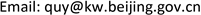DFT Studies on the Cohesive Energy of Graphene

Yan Qu

Beijing Advanced Materials and New Energy Technology Development Center, BeijingReceived: Dec. 11th, 2020; accepted: Jan. 11th, 2021; published: Jan. 18th, 2021ABSTRACT

In this paper, the geometric structure and cohesive energy of graphene are investigated under local density approximation (LDA) as well as generalized gradient approximation (GGA) based on first principles and density functional theory. Graphene is a hexagonal structure composed of carbon atoms. The total energy of the graphene system and the total energy of the isolated carbon atom system are calculated by the density functional theory. The calculation results show that the cohesive energy of graphene is about -9 to -10eV, and for different calculation methods and parameters, the cohesive energy obtained is always negative, which indicates that the graphene structure has lower energy relative to the isolated carbon atoms. Therefore, the attractive interaction makes the system more inclined to form the graphene structure compared to the isolated carbon atoms, indicating that the graphene structure is stable. This computational simulation study can provide a theoretical reference for the future development of graphene structures, as well as the performance studies and applications of novel structures (e.g., twist graphene).

Keywords:First Principles, Density Functional Theory, Graphene, Cohesive Energy1. 引言

2004年，英国学者Geim和Novoselov  发现他们可以用一种很简易的方式获得愈来愈薄的石墨薄片。他们通过从高定向热解石墨中剥离出石墨片，然后将薄片的两面粘在胶带上面，进一步撕开胶带从而将石墨一分为二。正是通过不断地这样操作，最终可以得到仅由一层碳原子构成的石墨烯结构。石墨烯是一种由碳原子组成六角型呈蜂巢晶格的二维材料 ，由于其具有优良的光学、力学、电学性能以及丰富的物理特性，在物理、材料学、加工、能源、医学等领域有着广阔的应用前景   ，近些年来一直是人们研究的热点。

2. 计算方法及过程2.1. 理论方法

E [ ρ ] = T 0 [ ρ ] + U e x t [ ρ ] + E c l [ ρ ] + E x c [ ρ ] (1)

ρ ( r ) = ∑ i = 1 N | ϕ i K S ( r ) | 2 (2)

[ − 1 2 ∇ i 2 + V ^ e f f ( r ) ] ϕ i K S ( r ) = ϵ i ϕ i K S ( r ) (3)

E [ ρ ] = ∑ i = 1 N ϵ i − 1 2 ∬ ρ ( r ) ρ ( r ′ ) | r − r ′ | d r d r ′ − ∫ V ^ x c ( r ) ρ ( r ) d r + E x c [ ρ ] (4)

∑ G ′ [ 1 2 | k + G ′ | 2 δ G G ′ + V k G G ′ e f f ] ϕ j ( G ′ ) = ϵ j ϕ j ( G ) (5)

E t o t = ∑ j ϵ j − Ω 1 2 ∑ G ≠ 0 V c o u l ( G ) ρ * ( G ) − Ω ∑ G ≠ 0 [ V x c ( G ) − ϵ x c ( G ) ] ρ * ( G )     + ∑ ν α ν ∑ μ Z μ + γ E w a l d (6)

ℏ 2 2 m | k + G | 2 ≤ E c u t o f f (7)

2.2. 计算石墨烯的几何结构

2.2. 计算过程及参数的选取2.2.1. 石墨烯体系计算

Parameters of graphene total energy calculatio

2.2.2. 孤立碳原子体系计算

Parameters of isolated carbon atom total energy calculatio

3. 计算结果和分析

Finalenergy , E = − 621.8196941698   eV (8)

Finalenergy , E = − 145.3495145913   eV (9)

E = − 621.8196941698   eV − ( − 4 × 145.3495145913   eV ) 4 ≈ − 10.105   eV (10)

Finalenergy , E = − 620.0817119187   eV (11)

E = − 620.0817119187   eV − ( − 4 × 145.3500139220   eV ) 4 ≈ − 9.670414   eV (12)

Calculation of the total energy of graphene under different parameters and method

1LDA280 eV10 * 6 * 1, 30USP−621.8197 eV
2LDA680 eV10 * 6 * 1, 30NCP−621.1066 eV
3GGA-PBE280 eV10 * 6 * 1, 30USP−621.0817 eV
4GGA-PBE680 eV10 * 6 * 1, 30NCP−621.4323 eV
5GGA-PBE750 eV10 * 6 * 1, 30NCP−621.4341 eV

Calculation of the total energy of isolated carbon atom under different parameters and method

1LDA280 eV1 * 1 * 1, 1USP−145.3495 eV
2LDA680 eV1 * 1 * 1, 1NCP−145.2731 eV
3GGA-PBE280 eV1 * 1 * 1, 1USP−145.7861 eV
4GGA-PBE680 eV1 * 1 * 1, 1NCP−145.7210 eV

4. 结果分析与讨论